# AKT-09/HW1

Solve the following problems and submit them in class by October 13, 2009:

Problem 1. If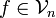$f \in {\mathcal V}_n$ and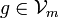$g \in {\mathcal V}_m$ then$f \cdot g \in {\mathcal V}_{n+m}$ (as what one would expect by looking at degrees of polynomials) and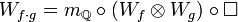$W_{f \cdot g} = m_\mathbb{Q} \circ (W_f \otimes W_g) \circ \Box$ where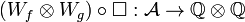$(W_f \otimes W_g) \circ \Box: {\mathcal A} \rightarrow \mathbb{Q} \otimes \mathbb{Q}$ and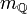$m_\mathbb{Q}$ is the multiplication of rationals. (See 090924-2, minute 36:01).

Problem 2. Let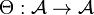$\Theta:{\mathcal A}\to{\mathcal A}$ be the multiplication operator by the 1-chord diagram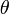$\theta$, and let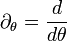$\partial_\theta=\frac{d}{d\theta}$ be the adjoint of multiplication by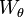$W_\theta$ on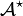${\mathcal A}^\star$, where$W_\theta$ is the obvious dual of$\theta$ in${\mathcal A}^\star$. Let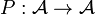$P:{\mathcal A}\to{\mathcal A}$ be defined by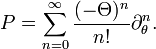$P = \sum_{n=0}^\infty \frac{(-\Theta)^n}{n!}\partial_\theta^n.$

Verify the following assertions, but submit only your work on assertions 4,5,7,11:

1.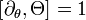$\left[\partial_\theta,\Theta\right]=1$, where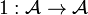$1:{\mathcal A}\to{\mathcal A}$ is the identity map and where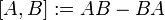$[A,B]:=AB-BA$ for any two operators.
2.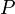$P$ is a degree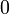$0$ operator; that is,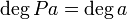$\deg Pa=\deg a$ for all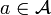$a\in{\mathcal A}$.
3.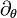$\partial_\theta$ satisfies Leibnitz' law: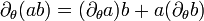$\partial_\theta(ab)=(\partial_\theta a)b+a(\partial_\theta b)$ for any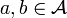$a,b\in{\mathcal A}$.
4.$P$ is an algebra morphism: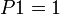$P1=1$ and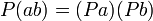$P(ab)=(Pa)(Pb)$.
5.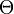$\Theta$ satisfies the co-Leibnitz law:$\Box\circ\Theta=(\Theta\otimes 1+1\otimes\Theta)\circ\Box$ (why does this deserve the name "the co-Leibnitz law"?).
6.$P$ is a co-algebra morphism: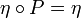$\eta\circ P=\eta$ (where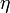$\eta$ is the co-unit of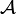${\mathcal A}$) and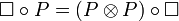$\Box\circ P=(P\otimes P)\circ\Box$.
7.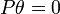$P\theta=0$ and hence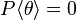$P\langle\theta\rangle=0$, where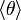$\langle\theta\rangle$ is the ideal generated by$\theta$ in the algebra${\mathcal A}$.
8. If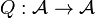$Q:{\mathcal A}\to{\mathcal A}$ is defined by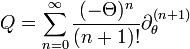$Q = \sum_{n=0}^\infty \frac{(-\Theta)^n}{(n+1)!}\partial_\theta^{(n+1)}$
then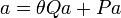$a=\theta Qa+Pa$ for all$a\in{\mathcal A}$.
9.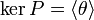$\ker P=\langle\theta\rangle$.
10.$P$ descends to a Hopf algebra morphism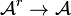${\mathcal A}^r\to{\mathcal A}$, and if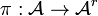$\pi:{\mathcal A}\to{\mathcal A}^r$ is the obvious projection, then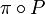$\pi\circ P$ is the identity of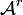${\mathcal A}^r$. (Recall that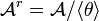${\mathcal A}^r={\mathcal A}/\langle\theta\rangle$).
11.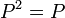$P^2=P$.

Idea for a good deed. Later than October 13, prepare a beautiful TeX writeup (including the motivation and all the details) of the solution of this assignment for publication on the web. For all I know this information in this form is not available elsewhere.

Mandatory but unenforced. Find yourself in the class photo and identify yourself as explained in the photo page.